Home Practice
For learners and parents For teachers and schools
Textbooks
Full catalogue
Pricing SupportLog in

We think you are located in United States. Is this correct?

# 20.4 Techniques of vector addition

## 20.4 Techniques of vector addition (ESAGP)

Now that you have learned about the mathematical properties of vectors, we return to vector addition in more detail. There are a number of techniques of vector addition. These techniques fall into two main categories - graphical and algebraic techniques.

### Graphical techniques (ESAGQ)

Graphical techniques involve drawing accurate scale diagrams to denote individual vectors and their resultants. We will look at just one graphical method: the head-to-tail method.

1. Draw a rough sketch of the situation.

2. Choose a scale and include a reference direction.

3. Choose any of the vectors and draw it as an arrow in the correct direction and of the correct length – remember to put an arrowhead on the end to denote its direction.

4. Take the next vector and draw it as an arrow starting from the arrowhead of the first vector in the correct direction and of the correct length.

5. Continue until you have drawn each vector – each time starting from the head of the previous vector. In this way, the vectors to be added are drawn one after the other head-to-tail.

6. The resultant is then the vector drawn from the tail of the first vector to the head of the last. Its magnitude can be determined from the length of its arrow using the scale. Its direction too can be determined from the scale diagram.

Let's consider some more examples of vector addition using displacements. The arrows tell you how far to move and in what direction. Arrows to the right correspond to steps forward, while arrows to the left correspond to steps backward. Look at all of the examples below and check them.

This example says $$\text{1}$$ step forward and then another step forward is the same as an arrow twice as long – two steps forward.

This example says $$\text{1}$$ step backward and then another step backward is the same as an arrow twice as long – two steps backward.

It is sometimes possible that you end up back where you started. In this case the net result of what you have done is that you have gone nowhere (your start and end points are at the same place). In this case, your resultant displacement is a vector with length zero units. We use the symbol $$\vec{0}$$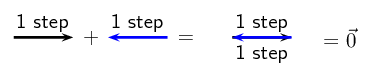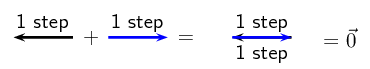Check the following examples in the same way. Arrows up the page can be seen as steps left and arrows down the page as steps right.

Try a couple to convince yourself!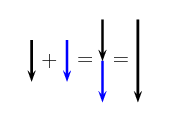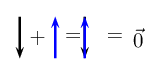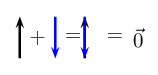It is important to realise that the directions are not special– forward and backwards' or left and right' are treated in the same way. The same is true of any set of parallel directions: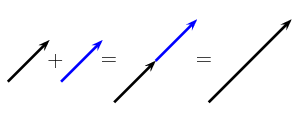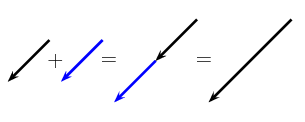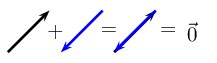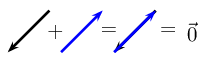In the above examples the separate displacements were parallel to one another. However the same head-to-tail technique of vector addition can be applied to vectors in any direction.

A car breaks down in the road and you and your friend, who happen to be walking past, help the driver push-start it. You and your friend stand together at the rear of the car. If you push with a force of $$\text{50}$$ $$\text{N}$$ and your friend pushes with a force of $$\text{45}$$ $$\text{N}$$, what is the resultant force on the car? Use the head-to-tail technique to calculate the answer graphically.

### Draw a rough sketch of the situation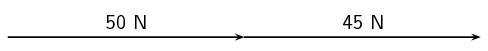### Choose a scale and a reference direction

Let's choose the direction to the right as the positive direction. The scale can be $$\text{1}$$ $$\text{cm}$$ = $$\text{10}$$ $$\text{N}$$.

### Choose one of the vectors and draw it as an arrow of the correct length in the correct direction

Start with your force vector and draw an arrow pointing to the right which is $$\text{5}$$ $$\text{cm}$$ long (i.e. $$\text{50}$$ $$\text{N}$$ = $$\text{5}$$ $$\times$$$$\text{10}$$ $$\text{N}$$, therefore, you must multiply your $$\text{cm}$$ scale by $$\text{5}$$ as well).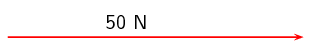### Take the next vector and draw it starting at the arrowhead of the previous vector.

Since your friend is pushing in the same direction as you, your force vectors must point in the same direction. Using the scale, this arrow should be $$\text{4,5}$$ $$\text{cm}$$ long.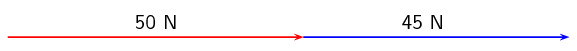### Draw the resultant, measure its length and find its direction

There are only two vectors in this problem, so the resultant vector must be drawn from the tail (i.e. starting point) of the first vector to the head of the second vector.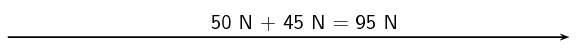The resultant vector measures $$\text{9,5}$$ $$\text{cm}$$ and points to the right. Therefore the resultant force must be $$\text{95}$$ $$\text{N}$$ in the positive direction (or to the right).

Use the graphical head-to-tail method to determine the resultant force on a rugby player if two players on his team are pushing him forwards with forces of $$\vec{{F}_{1}} = \text{60}\text{ N}$$ and $$\vec{{F}_{2}} = \text{90}\text{ N}$$ respectively and two players from the opposing team are pushing him backwards with forces of $$\vec{{F}_{3}} = \text{100}\text{ N}$$ and $$\vec{{F}_{4}} = \text{65}\text{ N}$$ respectively.

### Choose a scale and a reference direction

Let's choose a scale of $$\text{0,5}$$ $$\text{cm}$$= $$\text{10}$$ $$\text{N}$$ and for our diagram we will define the positive direction as to the right.

### Choose one of the vectors and draw it as an arrow of the correct length in the correct direction

We will start with drawing the vector $$\vec{{F}_{1}}= \text{60}\text{ N}$$, pointing in the positive direction. Using our scale of $$\text{0,5}$$ $$\text{cm}$$ = $$\text{10}$$ $$\text{N}$$, the length of the arrow must be $$\text{3}$$ $$\text{cm}$$ pointing to the right.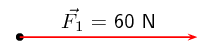### Take the next vector and draw it starting at the arrowhead of the previous vector

The next vector is $$\vec{{F}_{2}} = \text{90}\text{ N}$$ in the same direction as $$\vec{{F}_{1}}$$. Using the scale, the arrow should be $$\text{4,5}$$ $$\text{cm}$$ long and pointing to the right.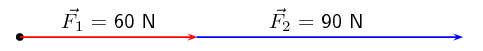### Take the next vector and draw it starting at the arrowhead of the previous vector

The next vector is $$\vec{{F}_{3}} = \text{100}\text{ N}$$ in the opposite direction. Using the scale, this arrow should be $$\text{5}$$ $$\text{cm}$$ long and point to the left.

Note: We are working in one dimension so this arrow would be drawn on top of the first vectors to the left. This will get messy so we'll draw it next to the actual line as well to show you what it looks like.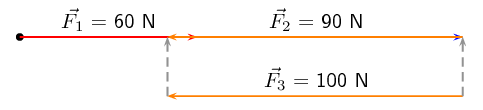### Take the next vector and draw it starting at the arrowhead of the previous vector

The fourth vector is $$\vec{{F}_{4}} = \text{65}\text{ N}$$ also in the opposite direction. Using the scale, this arrow must be $$\text{3,25}$$ $$\text{cm}$$ long and point to the left.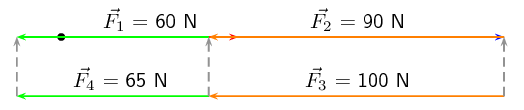### Draw the resultant, measure its length and find its direction

We have now drawn all the force vectors that are being applied to the player. The resultant vector is the arrow which starts at the tail of the first vector and ends at the head of the last drawn vector.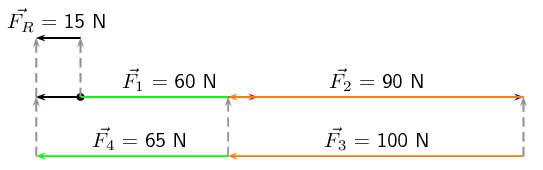The resultant vector measures $$\text{0,75}$$ $$\text{cm}$$ which, using our scale is equivalent to $$\text{15}$$ $$\text{N}$$ and points to the left (or the negative direction or the direction the opposing team members are pushing in).

temp text

### Algebraic techniques (ESAGR)

#### Vectors in a straight line

Whenever you are faced with adding vectors acting in a straight line (i.e. some directed left and some right, or some acting up and others down) you can use a very simple algebraic technique:

Method: Addition/Subtraction of Vectors in a Straight Line

1. Choose a positive direction. As an example, for situations involving displacements in the directions west and east, you might choose west as your positive direction. In that case, displacements east are negative.

2. Next simply add (or subtract) the magnitude of the vectors using the appropriate signs.

3. As a final step the direction of the resultant should be included in words (positive answers are in the positive direction, while negative resultants are in the negative direction).

Let us consider a few examples.

## Worked example 5: Adding vectors algebraically 1

A tennis ball is rolled towards a wall which is $$\text{10}$$ $$\text{m}$$ away from the ball. If after striking the wall the ball rolls a further $$\text{2,5}$$ $$\text{m}$$ along the ground away from the wall, calculate algebraically the ball's resultant displacement.

### Draw a rough sketch of the situation### Decide which method to use to calculate the resultant

We know that the resultant displacement of the ball ($$\vec{x_{R}}$$) is equal to the sum of the ball's separate displacements ($$\vec{x_{1}}$$ and $$\vec{x_{2}}$$):

$\vec{x_{R}} = \vec{x_{1}} + \vec{x_{2}}$

Since the motion of the ball is in a straight line (i.e. the ball moves towards and away from the wall), we can use the method of algebraic addition just explained.

### Choose a positive direction

Let's choose the positive direction to be towards the wall. This means that the negative direction is away from the wall.

### Now define our vectors algebraically

With right positive:

\begin{align*} \vec{x_{1}} & = + \text{10,0}\text{ m} \\ \vec{x_{2}} & = -\text{2,5}\text{ m} \end{align*}

Next we simply add the two displacements to give the resultant:

\begin{align*} \vec{x_{R}} & = \left(+\text{10,0}\text{ m}\right) + \left(-\text{2,5}\text{ m}\right) \\ & = \left(+\text{7,5}\text{ m}\right) \end{align*}

### Quote the resultant

Finally, in this case towards the wall is the positive direction, so: $$\vec{x_{R}} = \text{7,5}\text{ m}$$ towards the wall.

## Worked example 6: Subtracting vectors algebraically 1

Suppose that a tennis ball is thrown horizontally towards a wall at an initial velocity of $$\text{3}$$ $$\text{m·s^{-1}}$$ to the right. After striking the wall, the ball returns to the thrower at $$\text{2}$$ $$\text{m·s^{-1}}$$. Determine the change in velocity of the ball.

### Draw a sketch

A quick sketch will help us understand the problem.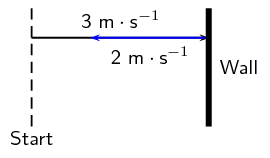### Decide which method to use to calculate the resultant

Remember that velocity is a vector. The change in the velocity of the ball is equal to the difference between the ball's initial and final velocities:

$\Delta \vec{v} = \vec{v_{f}} - \vec{v_{i}}$

Since the ball moves along a straight line (i.e. left and right), we can use the algebraic technique of vector subtraction just discussed.

### Choose a positive direction

Choose the positive direction to be towards the wall. This means that the negative direction is away from the wall.

### Now define our vectors algebraically

\begin{align*} \vec{v_{i}} & = +\text{3}\text{ m·s$^{-1}$} \\ \vec{v_{f}} & = -\text{2}\text{ m·s$^{-1}$} \end{align*}

### Subtract the vectors

Thus, the change in velocity of the ball is:

\begin{align*} \Delta \vec{v} & = \left(-\text{2}\text{ m·s$^{-1}$}\right) - \left(+\text{3}\text{ m·s$^{-1}$}\right) \\ & = -\text{5}\text{ m·s$^{-1}$} \end{align*}

### Quote the resultant

Remember that in this case towards the wall means a positive velocity,

so away from the wall means a negative velocity: $$\Delta \vec{v}=\text{5}\text{ m·s^{-1}}$$ away from the wall.

## Worked example 7: Adding vectors algebraically 2

A man applies a force of $$\text{5}$$ $$\text{N}$$ on a crate. The crate pushes back on the man with a force of $$\text{2}$$ $$\text{N}$$. Calculate algebraically the resultant force that the man applies to the crate.

### Draw a sketch

A quick sketch will help us understand the problem.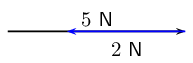### Decide which method to use to calculate the resultant

Remember that force is a vector. Since the crate moves along a straight line (i.e. left and right), we can use the algebraic technique of vector addition just discussed.

### Choose a positive direction

Choose the positive direction to be towards the crate (i.e. in the same direction that the man is pushing). This means that the negative direction is away from the crate (i.e. against the direction that the man is pushing).

### Now define our vectors algebraically

\begin{align*} \vec{F_{\text{man}}} & = +\text{5}\text{ N} \\ \vec{F_{\text{crate}}} & = -\text{2}\text{ N} \end{align*}

### Subtract the vectors

Thus, the resultant force is:

\begin{align*} \vec{F_{\text{man}}} + \vec{F_{\text{crate}}} & = \left(\text{5}\text{ N}\right) + \left(\text{2}\text{ N}\right) \\ & = \text{7}\text{ N} \end{align*}

### Quote the resultant

Remember that in this case towards the crate means a positive force: $$\text{7}$$ $$\text{N}$$ towards the crate.

Remember that the technique of addition and subtraction just discussed can only be applied to vectors acting along a straight line. When vectors are not in a straight line, i.e. at an angle to each other then simple geometric and trigonometric techniques can be used to find resultant vectors.

## Resultant Vectors

Textbook Exercise 20.4

Harold walks to school by walking $$\text{600}$$ $$\text{m}$$ Northeast and then $$\text{500}$$ $$\text{m}$$ N $$\text{40}$$$$\text{°}$$ W. Determine his resultant displacement by using accurate scale drawings.

Solution not yet available

A dove flies from her nest, looking for food for her chick. She flies at a velocity of $$\text{2}$$ $$\text{m·s^{-1}}$$ on a bearing of $$\text{135}$$$$\text{°}$$ and then at a velocity of $$\text{1,2}$$ $$\text{m·s^{-1}}$$ on a bearing of $$\text{230}$$$$\text{°}$$. Calculate her resultant velocity by using accurate scale drawings.

Solution not yet available

A squash ball is dropped to the floor with an initial velocity of $$\text{2,5}$$ $$\text{m·s^{-1}}$$. It rebounds (comes back up) with a velocity of $$\text{0,5}$$ $$\text{m·s^{-1}}$$.

1. What is the change in velocity of the squash ball?

2. What is the resultant velocity of the squash ball?

Solution not yet available

A frog is trying to cross a river. It swims at $$\text{3}$$ $$\text{m·s^{-1}}$$ in a northerly direction towards the opposite bank. The water is flowing in a westerly direction at $$\text{5}$$ $$\text{m·s^{-1}}$$. Find the frog's resultant velocity by using appropriate calculations. Include a rough sketch of the situation in your answer.

Solution not yet available

Mpihlonhle walks to the shop by walking $$\text{500}$$ $$\text{m}$$ Northwest and then $$\text{400}$$ $$\text{m}$$ N $$\text{30}$$$$\text{°}$$ E. Determine her resultant displacement by doing appropriate calculations.

Solution not yet available